## The Matrix Representation of Operators and Wavefunctions

We will define our vectors and matrices using a complete set of, orthonormal basis states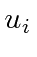, usually the set of eigenfunctions of a Hermitian operator. These basis states are analogous to the orthonormal unit vectors in Euclidean space.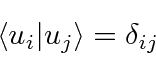Define the components of a state vector(analogous to).The wavefunctions are therefore represented as vectors.Define the matrix elementWe know that an operator acting on a wavefunction gives a wavefunction.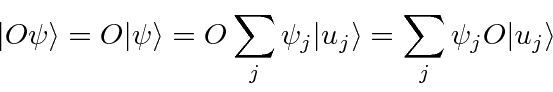If we dotinto this equation from the left, we getThis is exactly the formula for a state vector equals a matrix operator times a state vector.Similarly, we can look at the product of two operators (using the identity).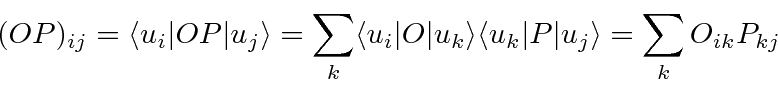This is exactly the formula for the product of two matrices.So, wave functions are represented by vectors and operators by matrices, all in the space of orthonormal functions.

* Example: The Harmonic Oscillator Hamiltonian Matrix.*
* Example: The harmonic oscillator raising operator.*
* Example: The harmonic oscillator lowering operator.*

Now compute the matrix for the Hermitian Conjugate of an operator.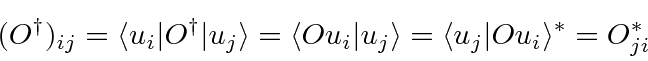The Hermitian Conjugate matrix is the (complex) conjugate transpose.

Check that this is true forand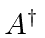.

We know that there is a difference between a bra vector and a ket vector. This becomes explicit in the matrix representation. If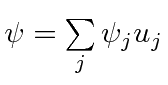and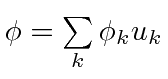then, the dot product isWe can write this in dot product in matrix notation asThe bra vector is the conjugate transpose of the ket vector. The both represent the same state but are different mathematical objects.

Jim Branson 2013-04-22